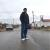## 2010 = 1+2-(3-4-5)*6*7*8-9

crazyinsomnia 发布于 2010/02/04 22:37

2010 = 1+2-(3-4-5)*6*7*8-9
2010 = 1-(2+(3-4-5)*6*7)*8+9
2010 = 1+2+(3+4*(5+6*7+8))*9
2010 = 1+2*(3*4*(5+6)-7)*8+9
2010 = 1*2*3*(4*(5*6+7*8)-9)
2010 = 1+2+(3+4*(5-6+7*8))*9
2010 = (1-2-3+4*(5/6+7*8))*9
2010 = (1+2+3*4)*(5-6+(7+8)*9)
2010 = 1+2+((3*(4+5)+6)*7-8)*9
2010 = (1+2+3)*(4*(5*6+7*8)-9)
2010 = 1+2+3*(4*(5+6)*(7+8)+9)
2010 = (1*2/3)*((4+5)*6*7*8-9)
2010 = (1-2-3)*((4+5)/6-7*8*9)
2010 = (1*2+(3-4*(5/6-7))*8)*9
2010 = 1*(2+(3-4*(5/6-7))*8)*9
2010 = (1+2*(3+4))*(5-6+(7+8)*9)

2010 = 12*34*5-6-7-8-9
2010 = 12*34*5+6*7-8*9
2010 = 1+2345*6/7+8-9
2010 = 12-3*(4+5-678)-9
2010 = 123*4*5-(6*7+8)*9
2010 = 1+2-3*(4*5*6-789)
2010 = 123*4*5+(6-7*8)*9
2010 = 12*34+(5+6+7)*89
2010 = 12*3*45+6*(7*8+9)
2010 = 12+3*(4-5+67+8)*9
2010 = (12-3)*4*56-7-8+9
2010 = 12-(3-4*56+7-8)*9
2010 = (1*2+34)*56-7-8+9
2010 = 1*(2+34)*56-7-8+9
2010 = 12*3*45-6*(7-8*9)
2010 = 1+2*(34*5*6-7)-8-9
2010 = 1+2-(3-45+6)*7*8-9
2010 = 1*2*3*(45*6+7*8+9)
2010 = (1+2)*3*4*56-7-8+9

..................................

00
l